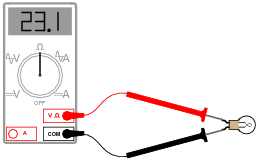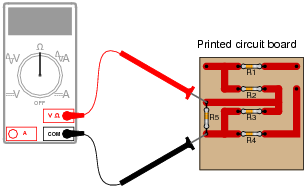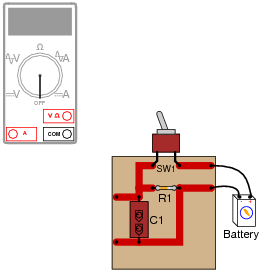# Basic Ohmmeter Use

## Basic Electricity

• #### Question 1

Explain how an ohmmeter is able to measure the resistance of a component (in this case, a light bulb) when there is no battery or other source of power connected to it:Also, identify the reading you would expect the ohmmeter to indicate if the light bulb were burnt out (failed “open”).

• #### Question 2

What would be wrong about measuring the resistance of a light bulb while it is being powered by a battery?• #### Question 3

Suppose we needed to determine the resistance of the light bulb in this circuit, while energized:Now, we know we cannot simply connect an ohmmeter to an energized circuit, so how is it possible to obtain the resistance measurement we desire?

• #### Question 4

Suppose a technician attempted to measure the resistance of resistor R5 on this printed circuit board:What is wrong with doing this?

• #### Question 5

Shown here is a circuit constructed on a PCB (a “Printed Circuit Board”), with copper “traces” serving as wires to connect the components together:How would the multimeter be used to measure the resistance of the component labeled “R1”? Include these important points in your answer:

The configuration of the multimeter (selector switch position, test lead jacks)
The connections of the meter test leads to the circuit
The state of the switch on the PCB (open or closed)
• #### Question 6

A technician picks up a resistor with the following color bands:

Color code: Brn, Blk, Sil, Gld

Having forgotten the resistor color code, and being too lazy to research the color code in a book, the technician decides to simply measure its resistance with an ohmmeter. The value this technician obtains is 0.6 Ω.

What is wrong with the technician’s measurement?

• #### Question 7

A technician picks up a resistor with the following color bands:

Color code: Org, Wht, Blu, Gld

Having forgotten the resistor color code, and being too lazy to research the color code in a book, the technician decides to simply measure its resistance with an ohmmeter. Holding one lead of the resistor and one test lead of the ohmmeter between the thumb and index finger of the left hand, and the other resistor lead and meter test lead between the thumb and index finger of the right hand (to keep each test lead of the meter in firm contact with the respective leads of the resistor), the technician obtains a resistance measurement of 1.5 MΩ.

What is wrong with the technician’s measurement?

• #### Question 8

What is a megger, and what type of resistance measurements would it typically be used for? Explain how the design of a “megger” differs from that of a normal ohmmeter.

Also, explain how improper use of a “megger” may actually be hazardous, either to the operator of the meter or to the circuit under test.

• #### Question 9

High-precision ohmmeters use a four-wire electrical connection to the resistance under test, rather than a two-wire connection. Explain how the four-wire system works, and why it provides better accuracy than two-wire resistance measurement.